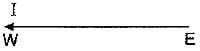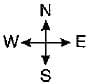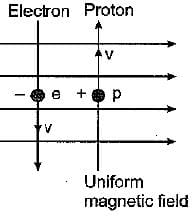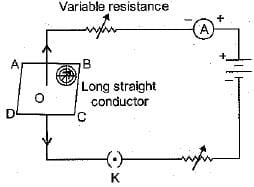# Magnetic Effects Of Current - 2

## 20 Questions MCQ Test Science & Technology for UPSC CSE | Magnetic Effects Of Current - 2

Description
Attempt Magnetic Effects Of Current - 2 | 20 questions in 20 minutes | Mock test for Class 10 preparation | Free important questions MCQ to study Science & Technology for UPSC CSE for Class 10 Exam | Download free PDF with solutions
QUESTION: 1

### The area around a magnet, in which its influence (force of attraction or repulsion) can be felt, is called its

Solution:

The region around a magnet, in which its influence can be felt, is called its magnetic field

QUESTION: 2

Solution:
QUESTION: 3

### A constant current I flows through a horizontal metal wire in the plane of the paper from east to west as shown in the figure. The direction of magnetic field will be from north to south at a point :Solution:

Let a constant current I flows through a horizontal wire from east to west. The direction of magnetic field, as per right-hand thumb rule, will be from north to south at a point directly below the current-carrying wire.

QUESTION: 4

What is that instrument which can detect the presence of electric current in a circuit?

Solution:
QUESTION: 5

The direction of magnetic field developed around a current-carrying conductor can be easily found by the use of

Solution:

The direction of magnetic field developed around a current-carrying conductor can be found by the use of right-hand thumb rule.

QUESTION: 6

Who has stated the Right hand Thumb Rule?

Solution:
QUESTION: 7

The strength of the magnetic field around a current-carrying straight conductor

Solution:

The strength of the magnetic field around a current-carrying straight conductor is directly proportional to the amount of current flowing in the conductor.

QUESTION: 8

What should be the core of an electromagnet?

Solution:
QUESTION: 9

The strength of a magnetic field inside a long current-carrying straight solenoid coil is

Solution:

The strength of a magnetic field inside a long, current-carrying, straight solenoid is uniform at all points. Moreover, the field depends on the amount of current flowing and the number of turns in solenoid coil besides its length

QUESTION: 10

The most important safety method used for protecting home appliances from short circuiting or overloading is

Solution:

It is most important method for protecting the electrical devices from short circuiting or overloading by stopping the flow of any large electric current exceeds from its rating.

QUESTION: 11

An electron moves with a speed v along positive direction of x-axis. If a magnetic field B acts along the positive y-direction, then the force on the electron will act along

Solution:

-ve  z axis

Explanation:

We use left hand rule or right hand palm rule or  cockscrew rule

as electron moves in positive x axis the current I is in -ve x axis

B is in +ve y axis

then -ve z axis is the direction of lorentz force

or u can just use vector cross product [-i x j ] = -k

QUESTION: 12

To convert an AC generator into DC generator

Solution:

To connect AC generator into DC generator, split ring type commutation must be used to get a unidirectional direct current.

QUESTION: 13

The strength of magnetic field along the axis of a solenoid coil :

Solution:

The strength of magnetic field along the axis of a solenoid coil increases on increasing the current flowing through the solenoid coil and on increasing the number of turns in the solenoid coil. Moreover, if a soft iron core is inserted inside the solenoid coil then magnetic field increases many fold.

QUESTION: 14

The strength of magnetic field inside a long current carrying straight solenoid is

Solution:

A current carrying solenoid produces a uniform magnetic field inside it.

QUESTION: 15

A current-carrying conductor is placed perpendicular to the direction of a uniform magnetic field. The direction of force acting on the conductor due to magnetic field is given by

Solution:

When a current-carrying straight conductor is placed perpendicular to the direction of a uniform magnetic field, it experiences a mechanical force. The direction of mechanical force is given by Fleming’s left-hand rule.

QUESTION: 16

Commercial electric motors do not use

Solution:

Using electromagnet, the magnetic field strength further increase by increasing the current. Hence it will enhance the power of electric.

QUESTION: 17

A uniform magnetic field exists in the plane of paper Electron Proton pointing from left to right as shown in the figure. In the field an electron and a proton move as shown. The electron and proton experienceSolution:

On applying Fleming’s left-hand rule we find that both electron as well as proton experience forces pointing into the plane of paper.

QUESTION: 18

For a current in a long straight solenoid N-pole and S-poIe are created at the two ends. Among the following statements, the incorrect statement is

Solution:

A solenoid behaves like a bar magnet. Hence the pattern of magnetic field associated with solenoid and around the bar magnet is same.

QUESTION: 19

Choose the wrong statement out of the following :

Solution:

Like magnetic poles (say N- and N-poles) always repel each other.

QUESTION: 20

If the key in the given arrangement is taken out (the circuit is made open) and magnetic field lines are drawn over the horizontal plane ABCD, the lines areSolution:

Magnetic field lines around a straight current carrying conductor are in the form of concentric circle.Use Code STAYHOME200 and get INR 200 additional OFF Use Coupon Code